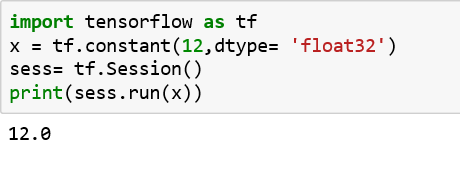top of page
Search
• Navin Manaswi

# Let's start working with TensorflowWhy Tensorflow?

You must be wondering if you need to know Tensorflow. Maybe you seek reasons to accept and adopt Tensorflow.

Since world is focusing on Artificial Intelligence in some way or other, Deep Learning (as the best component of Artificial Intelligence) is going to take the centre-stage. Deep Learning does a wonderful job in pattern recognition especially in the context of images, sound, speech, language and time series data

When we talk about Deep Learning, we're likely to discuss the best frameworks for development. Fortunately, in November 2015, Google has released Tensorflow, a Deep Learning framework, that has been used in most Google products such as Google search, spam detection, speech recognition, Google Allo, Google Now and Google Photos.

Tensorflow allows Model Parallelism and Data Parallelism. It provides multiple APIs, and the lowest level API --TensorFlow Core - provides you with complete programming control.

Tensors

The best way to start enjoying the Tensorflow library is to get comfortable with basic unit of data used in Tensorflow.

The basic unit is a Tensor. A Tensor is a mathematical object, a generalization of scalars, vectors and matrices.

Multidimensional array is a data structure suitable for representing a tensor

9 # a rank 0 tensor; this is a scalar with shape [ ]

[1. ,2., 3.] # a rank 1 tensor; this is a vector with shape 

[[5., 2., 7.], [3., 5., 4.]] # a rank 2 tensor; a matrix with shape [2, 3]

[[[6., 2., 3.]], [[7., 8., 9.]]] # a rank 3 tensor with shape [2, 1, 3]

Top 10 Key Points about Tensorflow

The core program of Tensorflow can be understood in simple statements:

1. Its programs are usually structured into a construction phase and execution phase.

2. The computational graph is built in construction phase

3. Construction phase assembles a graph having nodes (ops/operations) and edges (tensors)

4. Tensors as input and Tensors as output for any operation (node). Addition is an operation (node) that takes two tensors as input and gives a tensor as output

5. The computational graph is run in execution phase. Execution phase uses a session to execute ops in the graph

6. The simplest ops (operations) is a constant that takes no inputs but pass outputs to other ops that do computation

7. An example of ops can be multiplication (or addition, subtraction that takes two matrices as input and passes a matrix as output

8. The Tensorflow library has a default graph to which ops constructors add nodes

9. To actually evaluate the nodes, we must run the computational graph within a session

10. A session encapsulates the control and state of the TensorFlow runtime

TensorFlow programs use a Tensor data structure to represent all data -- only Tensors are passed between operations in the computation graph. You can think of a TensorFlow tensor as an n-dimensional array or list. A Tensor has a static type, a rank, and a shape.

Let's start coding in Tensorflow

There are three variable types in Tensorflow. Variable, Placeholder and Constant.

Variable:An explanation of the above code:

1. Import the Tensorflow module and call it tf

2. Create a constant value(x), and assign it the numerical value 12

3. Create a session for computing the values

4. Run just the variable x and print out its current value

To be comfortable, let's look at another example:An explanation of the above code:

1. Import the Tensorflow module and call it tf

2. Create a constant value called x, and give it the numerical value 12

3. Create a Variable called y, and define it as being the equation 12+11

4. Initialize the variables with tf.global_variables_initializer()

5. Create a session for computing the values

6. Run the model created in 4

7. Run just the variable y and print out its current value

Placeholder:

A placeholder is a variable that we can feed to at a later time. It is supposedly designed to accept external inputs. Placeholders can have one or multiple dimensions, meant for storing N-dimensional arraysAn explanation of the above code:

1. Import the Tensorflow module and call it tf

2. Create a placeholder called x, mentioning the float type

3. Create a Tensor called, y that is the operation of multiplying x by 10 and adding 500 to it. Note that any initial values for x is not defined.

4. Create a session for computing the values

5. Define the values of x in the feed_dict so as to run y

6. Print out its value

In the following example, we create a 2 by 4 matrix (2-D array) for storing some numbers in it. We then use the same operation as before to do element-wise multiplying by 10 and adding 1 to it.The first dimension of the placeholder is , that means any number of rows is allowed. We can also consider 2-D array in place of 1-D array.

Here is the code:Constant:

Constants are initialized when you call tf.constant, and their value can never change. By contrast, variables are not initialized when you call tf.Variable. To initialize all the variables in a TensorFlow program, you must explicitly call a special operation as follows:

It is important to realize init is a handle to the TensorFlow sub-graph that initializes all the global variables. Until we call sess.run, the variables are uninitialized.I hope you have enjoyed learning the basics of Tensorflow. You can find more details in my book to be published next month. Please feel free to comment.Navin Manaswi is writing a book on Deep Learning using Tensorflow. He has delivered end-to-end AI solutions for Telecom, Insurance, Digital Marketing and Smart City. He has worked for Dubai Smart City Project, General Mills and was also a part of a consulting firm in Malaysia. At present, he offers consultancy for Data Science and AI projects. He is an active AI blogger and author of many articles on Deep Learning and Machine Learning.